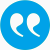#Kovacova, Gabriela, Rudloff, Birgit, Cialenco, Igor. 2022. Acceptability maximization. Frontiers of Mathematical Finance. 1 (2), 219-248.

BibTeX

## Abstract

The aim of this paper is to study the optimal investment problem by using coherent acceptability indices (CAIs) as a tool to measure the portfolio performance. We call this problem the acceptability maximization. First, we study the one-period (static) case, and propose a numerical algorithm that approximates the original problem by a sequence of risk minimization problems. The results are applied to several important CAIs, such as the gain-to-loss ratio, the risk-adjusted return on capital and the tail-value-at-risk based CAI. In the second part of the paper we investigate the acceptability maximization in a discrete time dynamic setup. Using robust representations of CAIs in terms of a family of dynamic coherent risk measures (DCRMs), we establish an intriguing dichotomy: if the corresponding family of DCRMs is recursive (i.e. strongly time consistent) and assuming some recursive structure of the market model, then the acceptability maximization problem reduces to just a one period problem and the maximal acceptability is constant across all states and times. On the other hand, if the family of DCRMs is not recursive, which is often the case, then the acceptability maximization problem ordinarily is a time-inconsistent stochastic control problem, similar to the classical mean-variance criteria. To overcome this form of time-inconsistency, we adapt to our setup the set-valued Bellman's principle recently proposed in  applied to two particular dynamic CAIs - the dynamic risk-adjusted return on capital and the dynamic gain-to-loss ratio. The obtained theoretical results are illustrated via numerical examples that include, in particular, the computation of the intermediate mean-risk efficient frontiers.

## Tags

Press 'enter' for creating the tag

## Publication's profile

Status of publication Published WU Journal article Frontiers of Mathematical Finance English Acceptability maximization 1 2 2022 219 248 Y http://dx.doi.org/10.3934/fmf.2021009 Y https://www.aimsciences.org/article/doi/10.3934/fmf.2021009

## Associations

People
Kovacova, Gabriela (Details)
Rudloff, Birgit (Details)
External
Cialenco, Igor (Illinois Institute of Technology, United States/USA)
Organization
Institute for Statistics and Mathematics IN (Details)
Research areas (ÖSTAT Classification 'Statistik Austria')
1118 Probability theory (Details)
1137 Financial mathematics (Details)
5361 Financial management (Details)